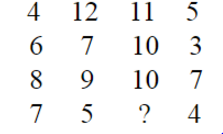# Series : 13 Expected Questions for the Exams#### Ques 1.

8,10,14,18,___, 34,50
a) 22
b) 26
c) 28
d) 30
Sol. Here are two alternate series, standing with the first two numbers, and each formed by doubling the preceding number in its own series and subtracting 2. (2*14=28;28-2=26). So, the answer is 26.

#### Ques 2.

2,6,12,20,30,?
a) 48
b) 46
c) 42
d) 40
Sol. here the series is 2,6,12,20,30,.....
⇒ 1×2,2×3,3×4,4×5,5×6,6×7,.......
So, the answer is (c) 42.

#### Ques 3.

0,4,18,48,100,?
Sol. 0,4,18,48,100,180
First term=1³-1²=0
Second term=2³-2²=4
Third term=3³-3²=18
Fourth term=4³-4²=48
Fifth term=5³-5²=100
Sixth term=6³-6²=180

#### Ques 4.

30, 68,130,222,?,520
a) 250
b) 350
c) 420
d) 480
Sol. The terms of the given series are 3³+3,4³+4,5³,+5,6³+6,7³+7,8³+8
∵ '?' is replaced by 350.

#### Ques 5.

93,86,79_, 65__
a) 58
b) 43
c) 51
d) 48
Sol. Here 93,93-7=86,86-7=79,79-7=72,72-7=65,65-7=58,58-7=51
∵ The answer is (c) 51.

#### Ques 6.

25, 22, 19, __, 13, 10, _?_
a) 4
b) 5
c) 6
d) 7
Sol. In this example sequence in the given series is -3.
∵ The answer is (d) 7.

#### Ques 7.

18, 4, 20, 6, 22, 8, 24, ___
a) 10
b) 26
c) 12
d) 28
Sol. Here alternate term is increased by 2.
∵ The answer is (a) 10.

#### Ques 8.

2, 2, __, 4,  6, __, 8, _?_
a) 6
b) 7
c) 8
d) 9
Sol. Here the series is like 2, 2, 4, 4, 6, 6, 8, 8
∵ The answer is (c) 8.

#### Ques 9.

a) 3
b) 4
c) 5
d) 6
Sol. Here each number in the bottom row is half the sum of the numbers in the other two rows. The sum of the numbers in the first and second rows. 7, 5 is 12, its half will be 6. So, the correct answer is 6.

#### Ques 10.a) 12
b) 14
c) 13
d) 8

Sol. 4+12=11+5
6+7=10+3
8+9=10+7
7+5=8+4
∵ The answer is (d) 8.

#### Ques 11.a) 2
b) 11
c) 3
d) 6
Sol. First row ÷ Second row × Third row = Fourth row
i.e. 6 ÷ 3 × 4 = 2×4=8
18÷2×3=9×3=27
15÷5×3=3×3=9
∵ The answer is (c) 3.

Sol. In each column, the product of the elements in the 1st and 2nd rows is equal to the product of elements in the 3rd and 4th rows.
∵ 4×8=32=2×16
6×10=60=5×12
12×6=72=9×8

#### Ques 13.a) 18/30
b) 20/30
c) 18/32
d) 19/32
Sol. The numbers at the top follow the sequence :-1,+2,-3,+4; those at the bottom : +1,-2,+3,-4
So the correct answer is (a) 18/30.#### What's trending in BankExamsToday

Smart Prep Kit for Banking Exams by Ramandeep Singh - Download here